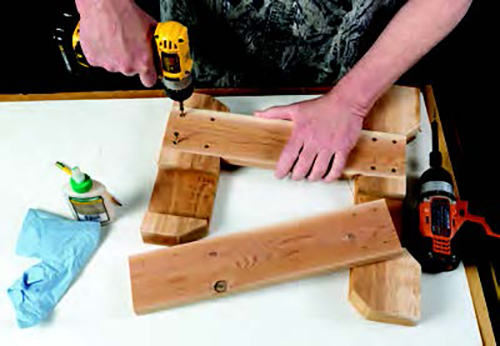# Easy Math Formula for Spacing FastenersSpacing fasteners at equal distances along the length of a workpiece, can be frustrating and time-consuming. Here’s a quick formula for calculating those distances. Decide the total number of fasteners you plan to install, at any given length. Add “one” to the fastener total and divide by the length of the workpiece.

For example: The length of a workpiece is 48″ and you decide to install five screws as fasteners. The formula is: 5 + 1 = 6. Dividing this total into the 48″ length, means the five screws should be spaced every 8 inches – at 8″, 16″, 24″, 32″ and 40″.

Also, if you want the first and last screws to be inset a certain distance from each end of the workpiece, subtract the total of these two distances from the workpiece length, first. Use the  decided first screw location as your starting point. Then apply the quick formula for placement of the remaining screws.

Depending on the length of the workpiece and/or the number of fasteners desired, you will sometimes end up with fractional measurements, but the math is still the same. A simple way to avoid the frustration of calculating fractions, is to use a metric tape for all measuring when using this formula.

– Robert W. Hicks